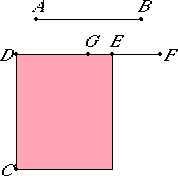# Proposition 111

The apotome is not the same with the binomial straight line.

Let AB be an apotome.

I say that AB is not the same with the binomial straight line.

If possible, let it be so. Set out a rational straight line DC, and to CD apply the rectangle CE equal to the square on AB and producing DE as breadth.

X.97

Then, since AB is an apotome, DE is a first apotome.X.Def.III.2

Let EF be the annex to it. Then DF and FE are rational straight lines commensurable in square only, the square on DF is greater than the square on FE by the square on a straight line commensurable with DF, and DF is commensurable in length with the rational straight line DC set out.

X.60

Again, since AB is binomial, therefore DE is a first binomial straight line.

X.Def.II.1

Divide it into its terms at G, and let DG be the greater term. Then DG and GE are rational straight lines commensurable in square only, the square on DG is greater than the square on GE by the square on a straight line commensurable with DG, and the greater term DG is commensurable in length with the rational straight line DC set out.

Therefore DF is also commensurable in length with DG. Therefore the remainder GF is also commensurable in length with DF.

X.13

But DF is incommensurable in length with EF, therefore FG is also incommensurable in length with EF.

X.73

Therefore GF and FE are rational straight lines commensurable in square only, therefore EG is an apotome. But it is also rational: which is impossible.

Therefore, the apotome is not the same with the binomial straight line.

Q.E.D.

# Remark

The apotome and the irrational straight lines following it are neither the same with the medial straight line nor with one another.
X.22

For the square on a medial straight line, if applied to a rational straight line, produces as breadth a straight line rational and incommensurable in length with that to which it is applied,

X.97

while the square on an apotome, if applied to a rational straight line, produces as breadth a first apotome,

X.98

the square on a first apotome of a medial straight line, if applied to a rational straight line, produces as breadth a second apotome,

X.99

the square on a second apotome of a medial straight line, if applied to a rational straight line, produces as breadth a third apotome,

X.100

the square on a minor straight line, if applied to a rational straight line, produces as breadth a fourth apotome,

X.101

the square on the straight line which produces with a rational area a medial whole, if applied to a rational straight line, produces as breadth a fifth apotome,

X.102

and the square on the straight line which produces with a medial area a medial whole, if applied to a rational straight line, produces as breadth a sixth apotome.

Since the said breadths differ from the first and from one another, from the first because it is rational, and from one another since they are not the same in order, it is clear that the irrational straight lines themselves also differ from one an other.

X.111

Since the apotome has been proved not to be the same as the binomial straight line,

but, if applied to a rational straight line, the straight lines following the apotome produce breadths, each according to its own order, apotomes, and those following the binomial straight line themselves also, according to their order, produce the binomials as breadths, therefore those following the apotome are different, and those following the binomial straight line are different, so that there are, in order, thirteen irrational straight lines in all:

Medial
Binomial
First bimedial
Second bimedial
Major
Side of a rational plus a medial area
Side of the sum of two medial areas
Apotome
First apotome of a medial straight line
Second apotome of a medial straight line
Minor
Producing with a rational area a medial whole
Producing with a medial area a medial whole

## Guide

This proposition is not used in the rest of the Elements.